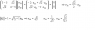# Learning e11 & e12: A Guide for Tuan

• tuanle007
In summary, someone needs to learn how to get eigenvalues and eigenvectors from a matrix. They found a way to do it yesterday.f

#### tuanle007

can someone look at the image...
can someone teach me/ help me remember how we can get
e11 and e12
thank you so much
tuan

here is the picture

#### Attachments

•untitled.PNG
1.5 KB · Views: 340
What is this; a homework question or what? I don't really understand what you want explaining to you. Is there a specific question that the attachment is a solution to?

You can get e11 and e12 as in the picture. Just write down the eigenvalue equation, multiply it out and solve the two given equations for the two unknowns. That's all that is happening. If you want more help, you need to be more specific.

Apparently this is to find eigenvectors of the matrix
$$\left(\begin{array}{cc} 3 & \sqrt{2} \\ \sqrt{2} & 4\end{array}\right)$$
corresponding to eigenvalue 5.

Since you want, by definition of "eigenvalue" and "eigenvector"
$$\left(\begin{array}{cc} 3 & \sqrt{2} \\ \sqrt{2} & 4\end{array}\right)\left(\begin{array}{c}e_{11} \\ e_{12}\end{array}\right)= \left(\begin{array}{c} 5e_{11} \\ 5e_{12}\end{array}\right)$$

$$\left(\begin{array}{cc}-2e_{11}+ \sqrt{2}e_{12}\\ \sqrt{2}e_{11}- e_{12}\end{array}\right)= \left(\begin{array}{c}0 \\ 0\end{array}\right)$$

That gives you the two equations $-2e_{11}+ \sqrt{2}e_{12}= 0$ and $\sqrt{2}e_{11}- e_{12}= 0$. Because 5 is an eigenvalue, if you try to solve those two simultaneous equations you will find they are "dependent"- they reduce to a single equation: $\sqrt{2}e_{11}= e_{12}$. Choosing either of those to be any number you want, you can solve for the other. In your attachment, they choose $e_{11}= 1$ so $e_{12}= \sqrt{2}$. Any eigenvector of the equation, corresponding to eigenvalue 5, is a multiple of $(1, \sqrt{2})$.

The other eigenvalue, by the way, is 2. What are the eigenvectors corresponding to eigenvalue 2?

In your attachment, they choose $e_{11}= 1$ so $e_{12}= \sqrt{2}$. Any eigenvector of the equation, corresponding to eigenvalue 5, is a multiple of $(1, \sqrt{2})$.
Actually, they choose $e_{11}, e_{12}$ such that the eigenvector has unit length
$$|| (e_{11}, e_{12}) || = \sqrt{ e_{11}^2 + e_{12}^2 }$$

i think compuchip is correct..
i found out how to do it yesterday..
u have to set (e11)^2 + (e12)^2 =1
and solve for e11, and e12...